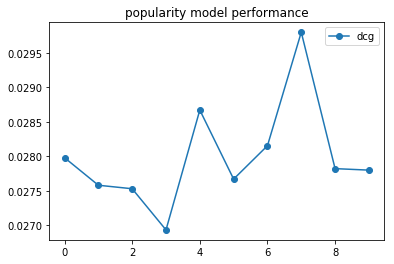# Five minute tutorial¶

In this tutorial we are going to learn the basic concepts of using Alpenglow by evaluating various baseline models on real world data.

## The data¶

We will use the dataset at http://info.ilab.sztaki.hu/~fbobee/alpenglow/alpenglow_sample_dataset. This is a processed version of the 30M dataset, where we

• only keep users above a certain activity threshold

• only keep the first events of listening sessions

• recode the items so they represent artists instead of tracks

Let’s start by importing standard packages and Alpenglow; and then reading the csv file using pandas. To avoid waiting too much for the experiments to complete, we limit the amount of records read to 200000.

```import pandas as pd
import matplotlib
import matplotlib.pyplot as plt
import alpenglow as ag

print(data.columns)
```

Output:

```Index(['time', 'user', 'item', 'score', 'eval', 'category'], dtype='object')
```

To run online experiments, you will need time-series data of user-item interactions in similar format to the above. The only required columns are the `‘user’` and `‘item’` columns – the rest will be autofilled if missing. The most important columns are the following:

• time: integer, the timestamp of the record. Controls various things, like evaluation timeframes or batch learning epochs. Defaults to `range(0,len(data))` if missing.

• user: integer, the user the activity belongs to. This column is required.

• item: integer, the item the activity belongs to. This column is required.

• score: double, the score corresponding to the given record. This could be for example the rating of the item in the case of explicit recommendation. Defaults to constant `1`.

• eval: boolean, whether to run ranking-evaluation on the record. Defaults to constant `True`.

## Our first model¶

Let’s start by evaluating a very basic model on the dataset, the popularity model. To do this, we need to import the preconfigured experiment from the package `alpenglow.experimens`.

```from alpenglow.experiments import PopularityExperiment
```

When creating an instance of the experiment, we can provide various configuration options and parameters.

```pop_experiment = PopularityExperiment(
top_k=100, # we are going to evaluate on top 100 ranking lists
seed=12345, # for reproducibility, we provide a random seed
)
```

You can see the list of the available options of online experiments in the documentation of `alpenglow.OnlineExperiment` and the parameters of this particular experiment in the documentation of the specific implementation (in this case `alpenglow.experiments.PopularityExperiment`) or, failing that, in the source code of the given class.

Running the experiment on the data is as simple as calling `run(data)`. Multiple options can be provided at this point, for a full list, refer to the documentation of `alpenglow.OnlineExperiment.OnlineExperiment.run()`.

```result = pop_experiment.run(data, verbose=True) #this might take a while
```

The `run()` method first builds the experiment out of C++ components according to the given parameters, then processes the data, training on it and evaluating the model at the same time. The returned object is a `pandas.DataFrame` object, which contains various information regarding the results of the experiment:

```print(result.columns)
```

Output:

```Index(['time', 'score', 'user', 'item', 'prediction', 'rank'], dtype='object')
```

Prediction is the score estimate given by the model and rank is the rank of the item in the toplist generated by the model. If the item is not on the toplist, rank is `NaN`.

The easiest way interpret the results is by using a predefined evaluator, for example `alpenglow.evaluation.DcgScore`:

```from alpenglow.evaluation import DcgScore
result['dcg'] = DcgScore(result)
```

The `DcgScore` class calculates the NDCG values for the given ranks and returns a `pandas.Series` object. This can be averaged and plotted easily to visualize the performance of the recommender model.

```daily_avg_dcg = result['dcg'].groupby((result['time']-result['time'].min())//86400).mean()
plt.plot(daily_avg_dcg,"o-", label="popularity")
plt.title('popularity model performance')
plt.legend()
```Putting it all together:

```import pandas as pd
import matplotlib
import matplotlib.pyplot as plt
from alpenglow.evaluation import DcgScore
from alpenglow.experiments import PopularityExperiment